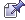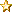January 29, 2020, 02:37:44 pm
 News: Giant crater may lie under Antarctic icehttp://space.newscientist.com/article/dn9268# THE ARABS INVENTED ZERO! - The Miracle Of Islamic Science

 Pages:    Go DownAuthor Topic: THE ARABS INVENTED ZERO! - The Miracle Of Islamic Science  (Read 2467 times)
Bianca
Superhero MemberPosts: 41646« on: May 10, 2009, 06:12:31 pm »

What is Taught: Isaac Newton, during the 17th century, discovered that white light consists of various rays of colored light.

What Should be Taught: This discovery was made in its entirety by al-Haytham (1lth century) and Kamal ad-Din (14th century). Newton did make original discoveries, but this was not one of them.

What is Taught: The concept of the finite nature of matter was first introduced by Antione Lavoisier during the 18th century. He discovered that, although matter may change its form or shape, its mass always remains the same. Thus, for instance, if water is heated to steam, if salt is dissolved in water or if a piece of wood is burned to ashes, the total mass remains unchanged.

What Should be Taught: The principles of this discovery were elaborated centuries before by Islamic Persia's great scholar, al-Biruni (d. 1050). Lavoisier was a disciple of the Muslim chemists and physicists and referred to their books frequently.

What is Taught: The Greeks were the developers of trigonometry.

What Should be Taught: Trigonometry remained largely a theoretical science among the Greeks. It was developed to a level of modern perfection by Muslim scholars, although the weight of the credit must be given to al-Battani. The words describing the basic functions of this science, sine, cosine and tangent, are all derived from Arabic terms. Thus, original contributions by the Greeks in trigonometry were minimal.

What is Taught: The use of decimal fractions in mathematics was first developed by a Dutchman, Simon Stevin, in 1589. He helped advance the mathematical sciences by replacing the cumbersome fractions, for instance, 1/2, with decimal fractions, for example, 0.5.

What Should be Taught: Muslim mathematicians were the first to utilize decimals instead of fractions on a large scale. Al-Kashi's book, Key to Arithmetic, was written at the beginning of the 15th century and was the stimulus for the systematic application of decimals to whole numbers and fractions thereof. It is highly probably that Stevin imported the idea to Europe from al-Kashi's work.

What is Taught: The first man to utilize algebraic symbols was the French mathematician, Francois Vieta. In 1591, he wrote an algebra book describing equations with letters such as the now familiar x and y's. Asimov says that this discovery had an impact similar to the progression from Roman numerals to Arabic numbers.

What Should be Taught: Muslim mathematicians, the inventors of algebra, introduced the concept of using letters for unknown variables in equations as early as the 9th century CE. Through this system, they solved a variety of complex equations, including quadratic and cubic equations. They used symbols to develop and perfect the binomial theorem.

What is Taught: The difficult cubic equations (x to the third power) remained unsolved until the 16th century when Niccolo Tartaglia, an Italian mathematician, solved them.

What Should be Taught: Cubic equations as well as numerous equations of even higher degrees were solved with ease by Muslim mathematicians as early as the 10th century.

What is Taught: The concept that numbers could be less than zero, that is negative numbers, was unknown until 1545 when Geronimo Cardano introduced the idea.

What Should he Taught: Muslim mathematicians introduced negative numbers for use in a variety of arithmetic functions at least 400 years prior to Cardano.
 Report SpamLogged

Your mind understands what you have been taught; your heart what is true.
 Pages:    Go Up